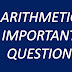QUEST FOR ARITHMETIC :: IBPS CLERK MAINS IMPORTANT TOPICS
Directions—(1–5) What approximate value will come in place of the question-mark (?) in the following questions ? (You are not expected to calculate the exact value.)

1. 465•84 + 765•86 – 211•99 = ?
(1) 1100
(2) 1080
(3) 1000
(4) 1020
(5) 1060

2. 151.1% of 151.1 + 151.1 = ?
(1) 380
(2) 400
(3) 350
(4) 420
(5) 440

3. 2001 × 473 ÷ 1001 – 245 = ?
(1) 650
(2) 700
(3) 950
(4) 850
(5)1000

4. √2450 - √1200 + √440 = ?
(1) 59
(2) 53
(3) 44
(4) 25
(5) 36

5. 501/58 * 291/101 ÷ 31/155 = ?
(1) 140
(2) 125
(3) 95
(4) 85
(5)110

6.If the compound interest on an amount of Rs. 29000 in two years is Rs. 9352.5, what is the rate of interest?
(1) 11
(2) 9
(3) 15
(4) 18
(5) None of these

7. Three friends A, B and C start running around a circular stadium and complete a single round in 8, 18 and 15 seconds respectively. After how many minutes will they meet again at the starting point for the first time?
(1) 12
(2) 6
(3) 8
(4) 15
(5) 18

8. The perimeter of a square is equal to the radius of a circle having area 39424 sq cm, what is the area of square?
(1) 1225 sq cm
(2) 441 sq cm
(3) 784 sq cm
(4) Can’t say
(5) None of these

Directions—(Q.9and 10) Study the following information carefully to answer the questions that follow—

A committee of five members is to be formed out of 5 Males, 6 Females and 3 Children. In how many different ways can it be done if—?

9. The committee should consist of 2 Males, 2 Females and 1 Child?
(1) 450
(2) 225
(3) 55
(4) 90
(5) None of these

10. The committee should include all the 3 Childs?
(1) 90
(2) 180
(3) 21
(4) 55
(5) None of these

EXPLANATION

1 . (4)

2. (1)

3. (2)

4. (5)

5. (2)
6. (3)
P = 29000 CI = 9352.5 N = 2 years  A = P + I = 38,352.5
Substituting the values in
A = P(1 + R/100)^n
Solving we get R = 15%.

7. (2)
The required time will be the LCM of 8, 18 and 15 which is 360 sec or 6 minutes.

8. (3)
Π R^2 = 39424
R = 112
Perimeter of square = 4a = 112
Side of square = 112/4 = 28
Area of square = 28^2 = 784 cm2.

9. (1)
Number of ways = 5C2 * 6C2 *3C1 = 450

10. (4)
Number of ways = 11C2 * 3C3 = 55.

QUEST FOR ARITHMETIC :: IBPS CLERK MAINS PRACTICE QUESTIONSReviewed by mani on 18:28:00 Rating: 5